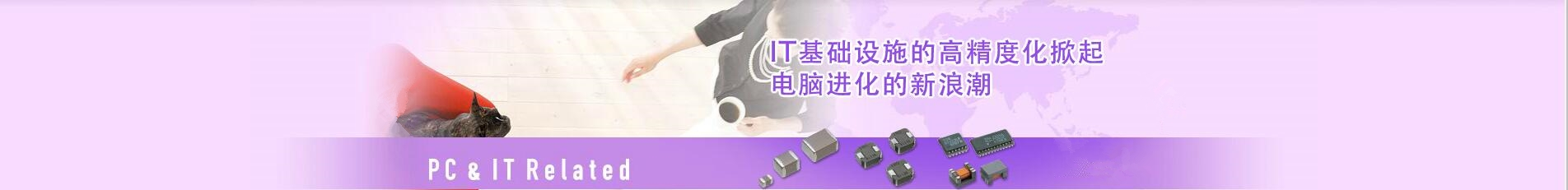## 日本TDK村田murata一级代理商-提供贴片电容器_电感器_蜂鸣器_滤波器_电阻磁珠_电源等产品代理服务

### 深圳市智成电子有限公司

Shenzhen Zhicheng Electronic Co., Ltd.

## 0755-23282269# 三星贴片电容型号规格对照表参数介绍

## 深圳智成电子有限公司（以下简称智成电子）教你快速读懂三星贴片电容型号规格对照表及相关参数介绍

1.系列编码
CL=积层陶瓷电容
2.尺寸编码

03-=0201 (0603 )         21=0805 (2012)         42=1808 ( 4520 )
05-0402 ( 1005 )          31=1206 ( 3216)        43-1812 ( 4532 )
10=0603 ( 1608)          32=1210 ( 3225)        55-2220 ( 5750)
3.电介质编码
I类                                         I1类
C- C0G S-S2H    L=S2L       A=X5R F=Y5V
P=P2H T-T2H                      B=X7R X=X6S
R=R2H U=U2J
4.电容编码

5.公差编码
B=±0.lpF           F=±lpF,±1%*          K=±10%
C=±0.25pF       G=±2%                     M=±20%
D=±0.5pF         J=±5%                    Z=+80/- -20%

6.额定电压编码
R=4V         O=16V            B=50V     E=250V      1=1000V
Q=6.3V      A=25V           C=100V    G=500V      J= 2000V
P=10V       L=35V            D=200V    H=630V      K=3000V
7.厚度编码
3-0.30毫米    A-0.65毫米    M=1.15毫米   1=2.00毫米    Q=1.25毫米*
5=0.50毫米  C-0.85毫米    F-1.25毫米    J=2.50毫米     V=2.50毫米*
8=0.80毫米  D=1.00毫米   H=1.60毫米   1=3.20毫米
8.内电极/端子/电镀编码
A=常规产品钯/银/镍屏蔽/锅100%
N=常规产品镍/铜/镍屏蘞/锡100%
G=常规产品铜/铜/镍屏蔽/锡100%
L=低侧而产品镍/锅/镍屏蔽/锡100%
9.产品编码
A=阵列(2元素)        L=LICC
B-=阵列(4-元素)     N=常规
C=高频                  Pa自动
10.特殊编码
N=预留给未来用途
11.包装编码
B=散装                                          0=纸板箱料带，10英寸料盘                                E=压花纸板箱，7英寸料盘
P=散装箱                                      D=纸板箱料带，13英寸料盘( 0000ea)                F=压花纸板箱，13英寸料盘
C=纸板箱料带，7英寸料盘            L =纸板箱料带，13英寸料盘( 15000ea )             S=压花纸板箱，10英寸料盘

1.因为三星贴片电容内部没有电解液，很适合高温下工作。智成电子的三星贴片电容的特点是寿命长、耐热、精确度高、滤高频谐波特性很好。

2.在电解电容器工作过程中，具备自动修复或阻隔氧化膜里的疵点所在性能，使氧化膜物质随时获得加固和恢复其应有的绝缘能力，而不致遭受连续的累积性破坏。这种独特自愈性能、确保了电容器产品的长寿命和稳定性的优点。

3.电解电容器具备非常高的工作场强，并较同类型电容器都大以此确保它微型化。

1、年Y代表2012，Z代表2013，A代表2014，B代表2015，下一年依次类推。

2、月份1~9，A代表10月，B代表11月,C代表12月

3、日期1~9，A代表10号,B代表11号……依次类推

A=自动化编码;D=年份;8=月份;Q=日份;O=产地;DF=投产日期序号、那么这就是2017年8月26日，年份字母代表有：X=2011年 Y=2012年 Z=2013年 A=2014年 B=2015年。

月份字母代表有：1=1月 2=2月 3=3月 4=4月以此类推到9月 A=10月 B=11月 C=12月。日份字母代表有：1=1日 2=2日 3=3日 4=4日 5=5日 6=6日 7=7日 8=8日9=9日 A=10日 B=11日 C=12日 以此类推。

产地字母代表有：K=(Korea HQ)韩国总部;M=(Mrxico)墨西哥;B=(Korea Busan)韩国釜山厂;O=(Dsem)东莞厂;P=(Philipping)菲律宾厂;S=(Soochow)苏州厂;T I J (Tsem)天津厂;T=(表示全程于天津厂制造);I=(表示抛光制造后于天津厂制造;J=(表示谨于天津厂)。

0755-23282269